Documentation

polaraxes

Create polar axes

Description

example

polaraxes creates the default polar axes in the current figure.

polaraxes(Name,Value) specifies properties for the PolarAxes object using one or more name-value pair arguments, for example, 'ThetaDir','clockwise'. For a list of properties, see PolarAxes Properties.

polaraxes(parent,___) creates the polar axes in the figure, panel, or tab specified by parent, instead of in the current figure. Use this option alone or with name-value pair arguments.

pax = polaraxes(___)returns the PolarAxes object created. Use pax to query and set properties of the PolarAxes object after it is created. For a list of properties, see PolarAxes Properties.

example

polaraxes(pax_in) makes the PolarAxes object pax_in the current axes.

Examples

collapse all

Create a new figure with polar axes and assign the polar axes object to pax. Add a plot to the axes. Then, use pax to modify axes properties.

figure
pax = polaraxes;
theta = 0:0.01:2*pi;
rho = sin(2*theta).*cos(2*theta);
polarplot(theta,rho)

pax.FontSize = 12;Create a figure with polar axes and assign the polar axes object to pax. Then, ensure pax is the current axes before calling the polarplot function.

figure
pax = polaraxes;

polaraxes(pax)
polarplot(1:10)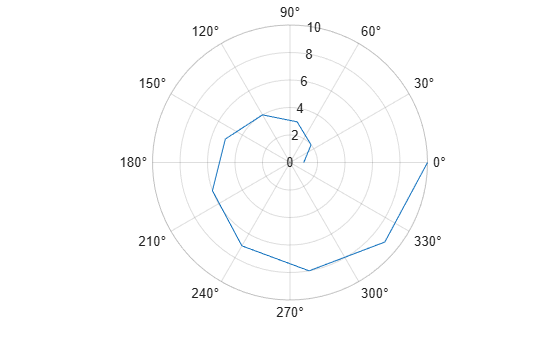Input Arguments

collapse all

Parent container, specified as a Figure, Panel, Tab, or TiledChartLayout object.

Polar axes to make current, specified as a PolarAxes object.

Name-Value Pair Arguments

Specify optional comma-separated pairs of Name,Value arguments. Name is the argument name and Value is the corresponding value. Name must appear inside quotes. You can specify several name and value pair arguments in any order as Name1,Value1,...,NameN,ValueN.

The properties listed here are only a subset. For a complete list, see PolarAxes Properties. Some graphics functions reset axes properties when plotting. To avoid graphics functions from overriding the property values, set axes properties after plotting.

Units for angle values, specified as one of these values:

• 'degrees' — Label the angles in degrees, and interpret the ThetaLim, ThetaTick, and RAxisLocation property values in degrees. When you switch the units from radians to degrees, MATLAB® converts the radian values in those three properties to the equivalent degree values.

• 'radians' — Label the angles in radians, and interpret the ThetaLim, ThetaTick, and RAxisLocation property values in radians. When you switch the units from degrees to radians, MATLAB converts the degree values in those three properties to the equivalent radian values.

Example: ax.ThetaAxisUnits = 'radians';

Location of the zero reference axis, specified as one of the values in this table.

ValueResult
'right'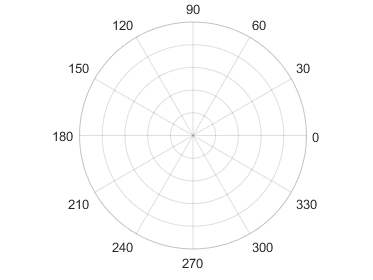'top'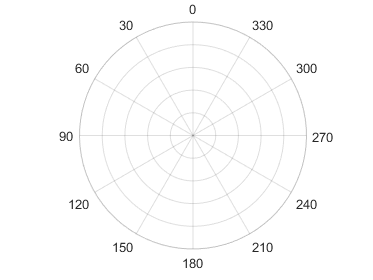'left'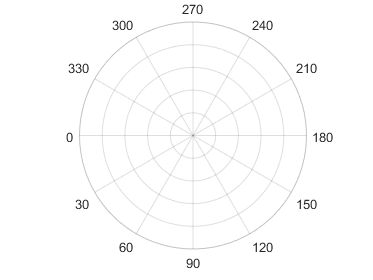'bottom'Example: ax.ThetaZeroLocation = 'left';

Direction of increasing angles, specified as one of the values in this table.

ValueResult
'counterclockwise'

Angles increase in a counterclockwise direction.'clockwise'

Angles increase in a clockwise direction.Example: ax.ThetaDir = 'clockwise';

Minimum and maximum angle values, specified as a two-element vector of the form [thmin thmax]. If the difference between the values is less than 360 degrees, then the theta-axis is a partial circle.

MATLAB interprets the values in units determined by the ThetaAxisUnits property.

Example: ax.ThetaLim = [0 180];

Angles at which to display lines extending from the origin, specified as a vector of increasing values. MATLAB labels the lines with the appropriate angle values, unless you specify different labels using the ThetaTickLabel property.

MATLAB interprets the values in units determined by the ThetaAxisUnits property.

Example: ax.ThetaTick = [0 90 180 270];

Alternatively, specify the values using the thetaticks function.

Color of the theta-axis, including the theta-axis grid lines, tick marks, tick labels. Specify this value as an RGB triplet, a hexadecimal color code, a color name, or a short name.

Note

If you specify the GridColor property, then the grid lines use the color in the GridColor property instead. See GridColorMode for more information.

For a custom color, specify an RGB triplet or a hexadecimal color code.

• An RGB triplet is a three-element row vector whose elements specify the intensities of the red, green, and blue components of the color. The intensities must be in the range [0,1]; for example, [0.4 0.6 0.7].

• A hexadecimal color code is a character vector or a string scalar that starts with a hash symbol (#) followed by three or six hexadecimal digits, which can range from 0 to F. The values are not case sensitive. Thus, the color codes '#FF8800', '#ff8800', '#F80', and '#f80' are equivalent.

Alternatively, you can specify some common colors by name. This table lists the named color options, the equivalent RGB triplets, and hexadecimal color codes.

Color NameShort NameRGB TripletHexadecimal Color CodeAppearance
'red''r'[1 0 0]'#FF0000''green''g'[0 1 0]'#00FF00''blue''b'[0 0 1]'#0000FF''cyan' 'c'[0 1 1]'#00FFFF''magenta''m'[1 0 1]'#FF00FF''yellow''y'[1 1 0]'#FFFF00''black''k'[0 0 0]'#000000''white''w'[1 1 1]'#FFFFFF''none'Not applicableNot applicableNot applicableNo color

Here are the RGB triplets and hexadecimal color codes for the default colors MATLAB uses in many types of plots.

RGB TripletHexadecimal Color CodeAppearance
[0 0.4470 0.7410]'#0072BD'[0.8500 0.3250 0.0980]'#D95319'[0.9290 0.6940 0.1250]'#EDB120'[0.4940 0.1840 0.5560]'#7E2F8E'[0.4660 0.6740 0.1880]'#77AC30'[0.3010 0.7450 0.9330]'#4DBEEE'[0.6350 0.0780 0.1840]'#A2142F'For example, ax.ThetaColor = 'r' changes the color to red.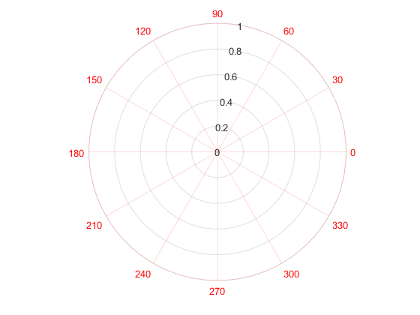Location of the r-axis, specified a scalar angle value. MATLAB interprets the values in units determined by the ThetaAxisUnits property.

Example: ax.RAxisLocation = 90;

Direction of increasing values along the r-axis, specified as one of these values:

• 'normal' — Values increase outward from the center of the chart. The radius at the origin corresponds to the minimum value stored in the RLim property (typically 0).

• 'reverse' — Values decrease outward from the center of the chart. The radius at the origin corresponds to the maximum value stored in the RLim property.

Example: ax.RDir = 'reverse';

Minimum and maximum radius limits, specified as a two-element vector of the form [rmin rmax], where rmax is a numeric value greater than rmin. You can specify both limits, or specify one limit and let the axes automatically calculate the other.

• To automatically set the minimum limit to the minimum data value, specify the first element as -inf, for example, [-inf 0].

• To automatically set the maximum limit to the maximum data value, specify the second element as inf, for example, [0 inf].

Alternatively, use the rlim function to set the limits.

Example: ax.RLim = [0 6];

Radius tick values, specified as a vector of increasing values. The radius tick values are the locations along the r-axis where the circular lines appear. The radius tick labels are the labels that you see next to each line. Use the RTickLabels property to specify the associated labels.

Example: ax.RTick = [0 2 4 6];

Alternatively, use the rticks function to specify the tick values.

Color of the r-axis, including the r-axis grid lines, tick marks, and tick labels. Specify this value as an RGB triplet, a hexadecimal color code, a color name, or a short name.

Note

If you specify the GridColor property, then the grid lines use the color in the GridColor property instead. See GridColorMode for more information.

For a custom color, specify an RGB triplet or a hexadecimal color code.

• An RGB triplet is a three-element row vector whose elements specify the intensities of the red, green, and blue components of the color. The intensities must be in the range [0,1]; for example, [0.4 0.6 0.7].

• A hexadecimal color code is a character vector or a string scalar that starts with a hash symbol (#) followed by three or six hexadecimal digits, which can range from 0 to F. The values are not case sensitive. Thus, the color codes '#FF8800', '#ff8800', '#F80', and '#f80' are equivalent.

Alternatively, you can specify some common colors by name. This table lists the named color options, the equivalent RGB triplets, and hexadecimal color codes.

Color NameShort NameRGB TripletHexadecimal Color CodeAppearance
'red''r'[1 0 0]'#FF0000''green''g'[0 1 0]'#00FF00''blue''b'[0 0 1]'#0000FF''cyan' 'c'[0 1 1]'#00FFFF''magenta''m'[1 0 1]'#FF00FF''yellow''y'[1 1 0]'#FFFF00''black''k'[0 0 0]'#000000''white''w'[1 1 1]'#FFFFFF''none'Not applicableNot applicableNot applicableNo color

Here are the RGB triplets and hexadecimal color codes for the default colors MATLAB uses in many types of plots.

RGB TripletHexadecimal Color CodeAppearance
[0 0.4470 0.7410]'#0072BD'[0.8500 0.3250 0.0980]'#D95319'[0.9290 0.6940 0.1250]'#EDB120'[0.4940 0.1840 0.5560]'#7E2F8E'[0.4660 0.6740 0.1880]'#77AC30'[0.3010 0.7450 0.9330]'#4DBEEE'[0.6350 0.0780 0.1840]'#A2142F'For example, ax.RColor = 'r' changes the color to red.